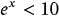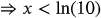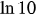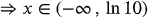1
62
views
53a
Problem

Problem 53a

Chapter 1: Functions and ModelsTextbook ExpertVerified Tutor
14 Oct 2021

Given information

Given equation is.

Step-by-step explanation

Step 1.

On simplification,Therefore the solutions of the given inequality is the set of all real numbers less than. That is,